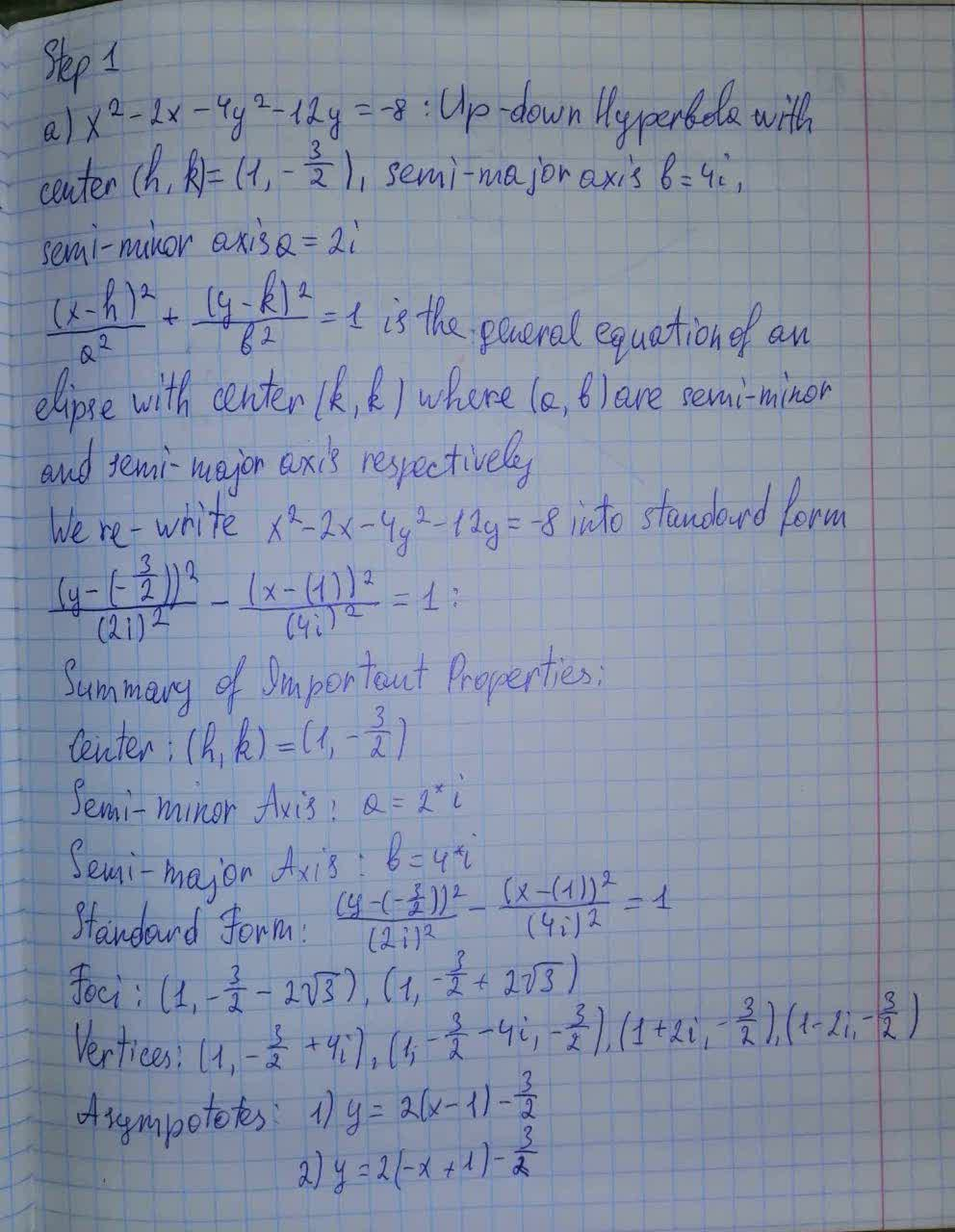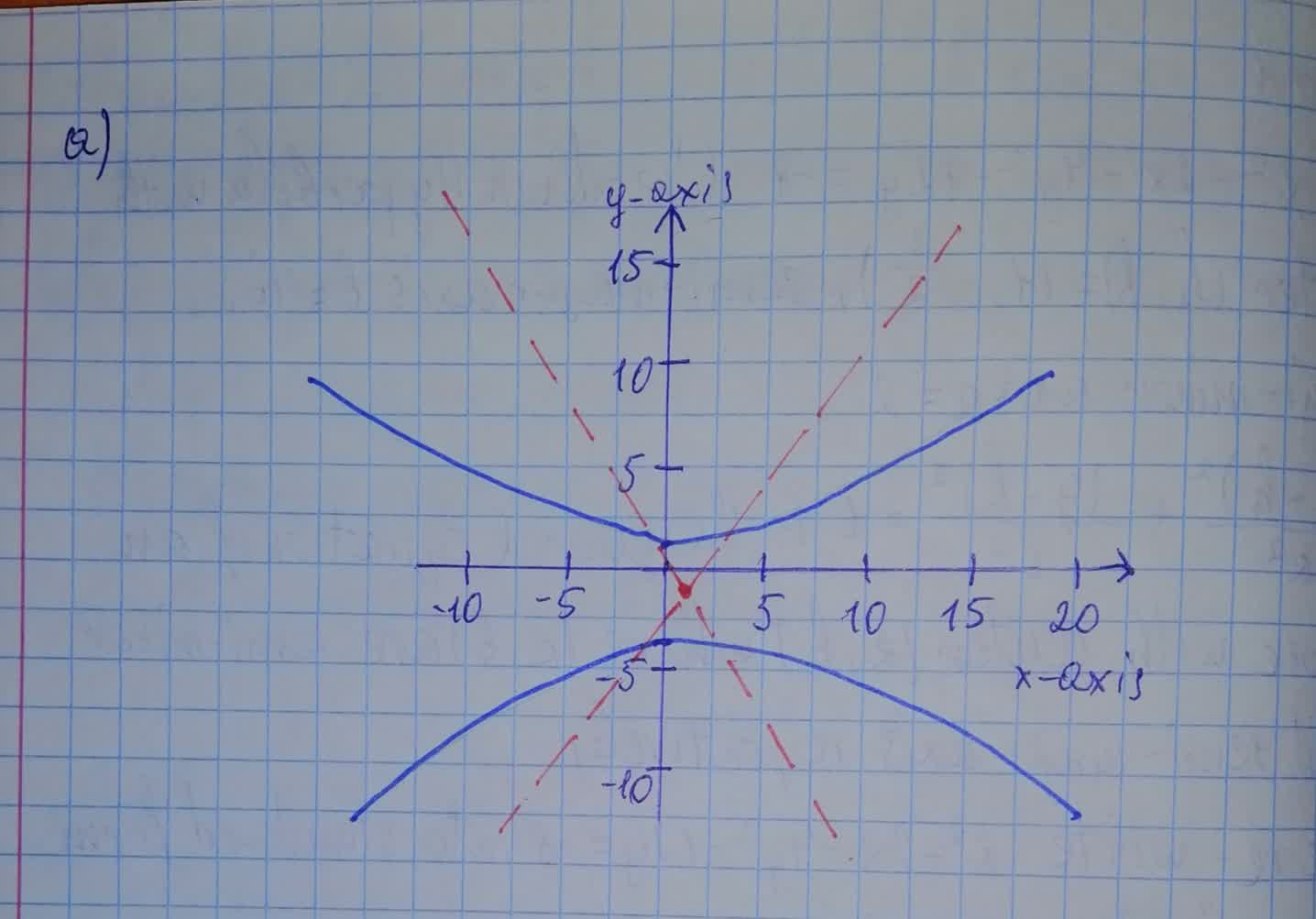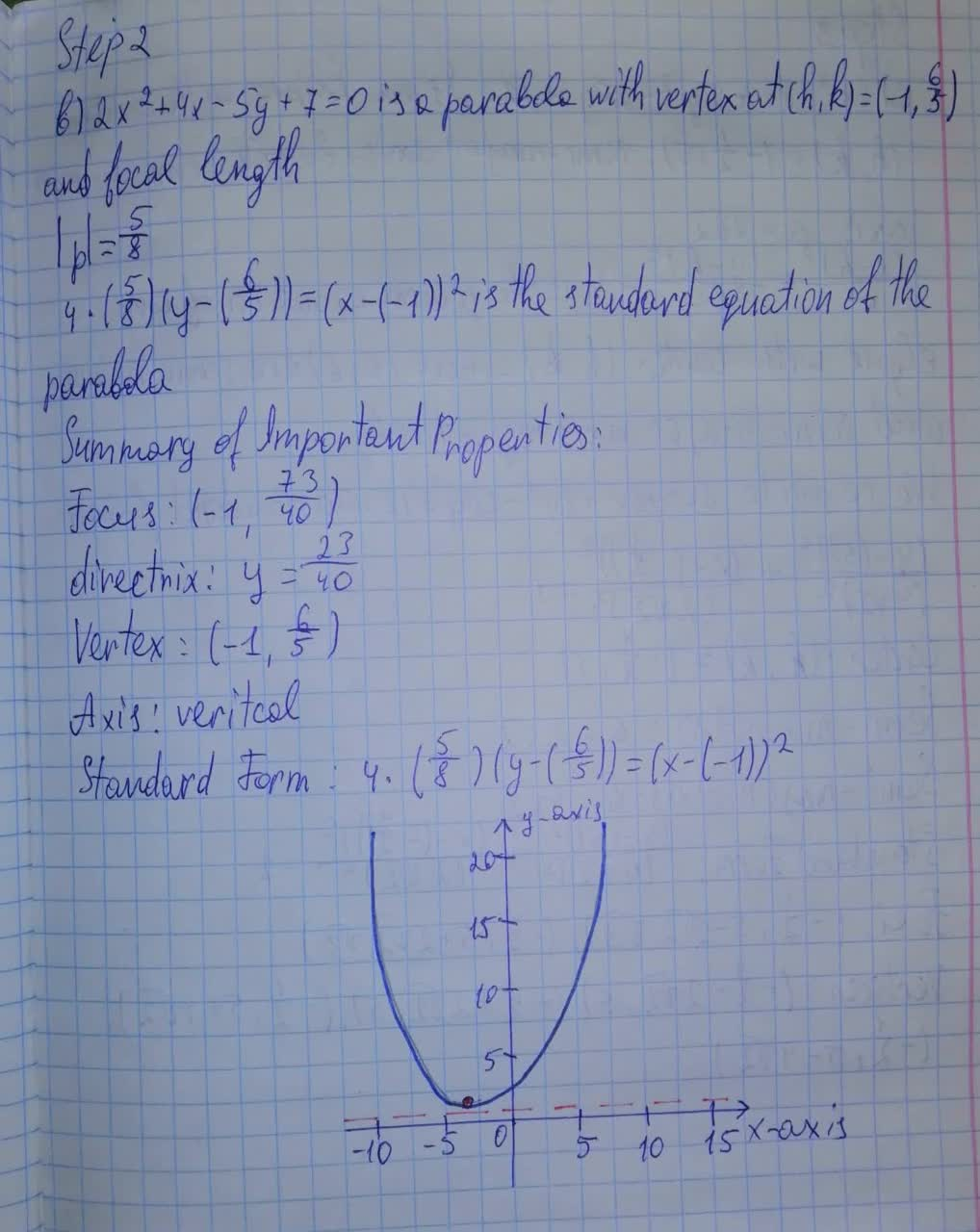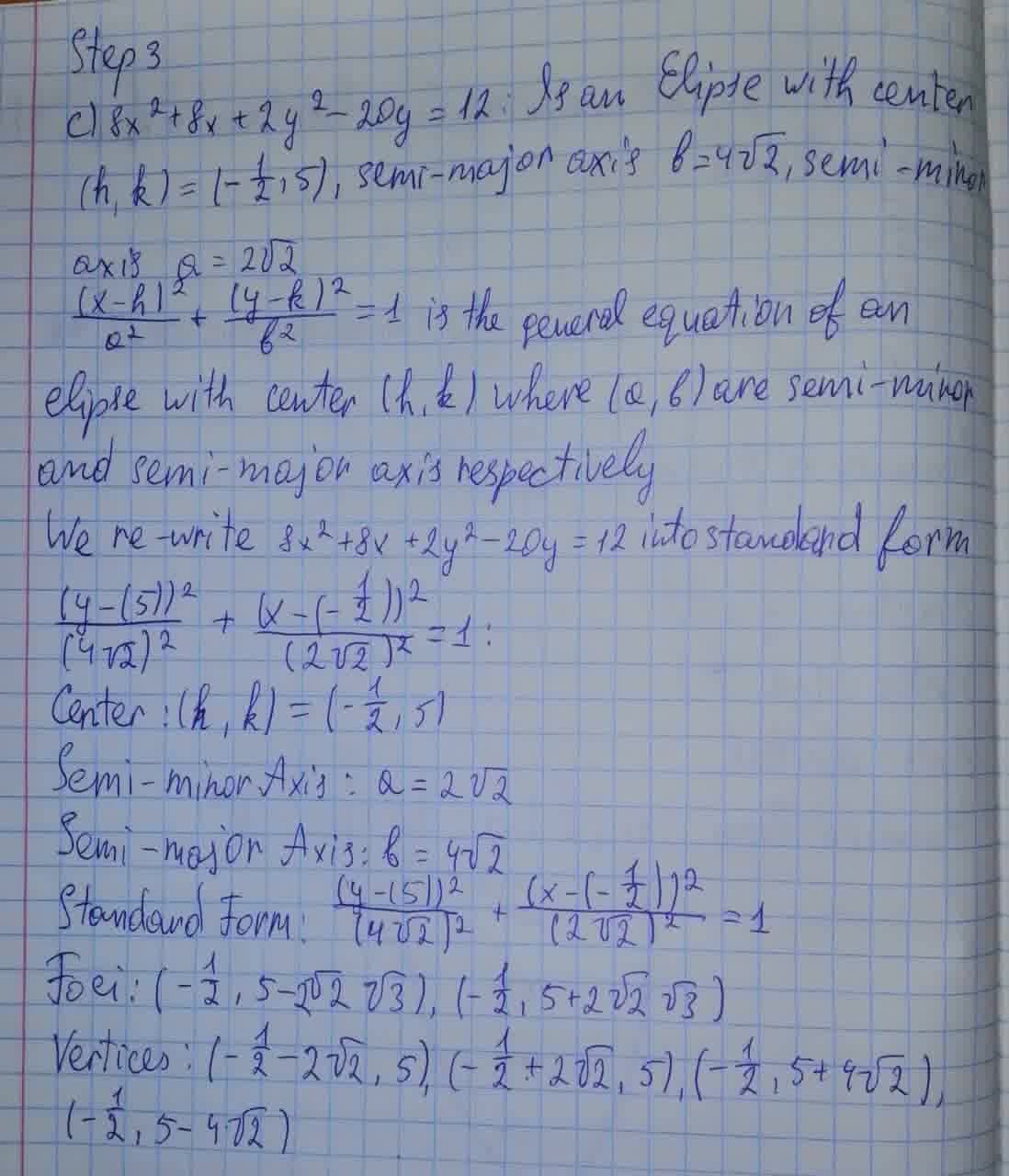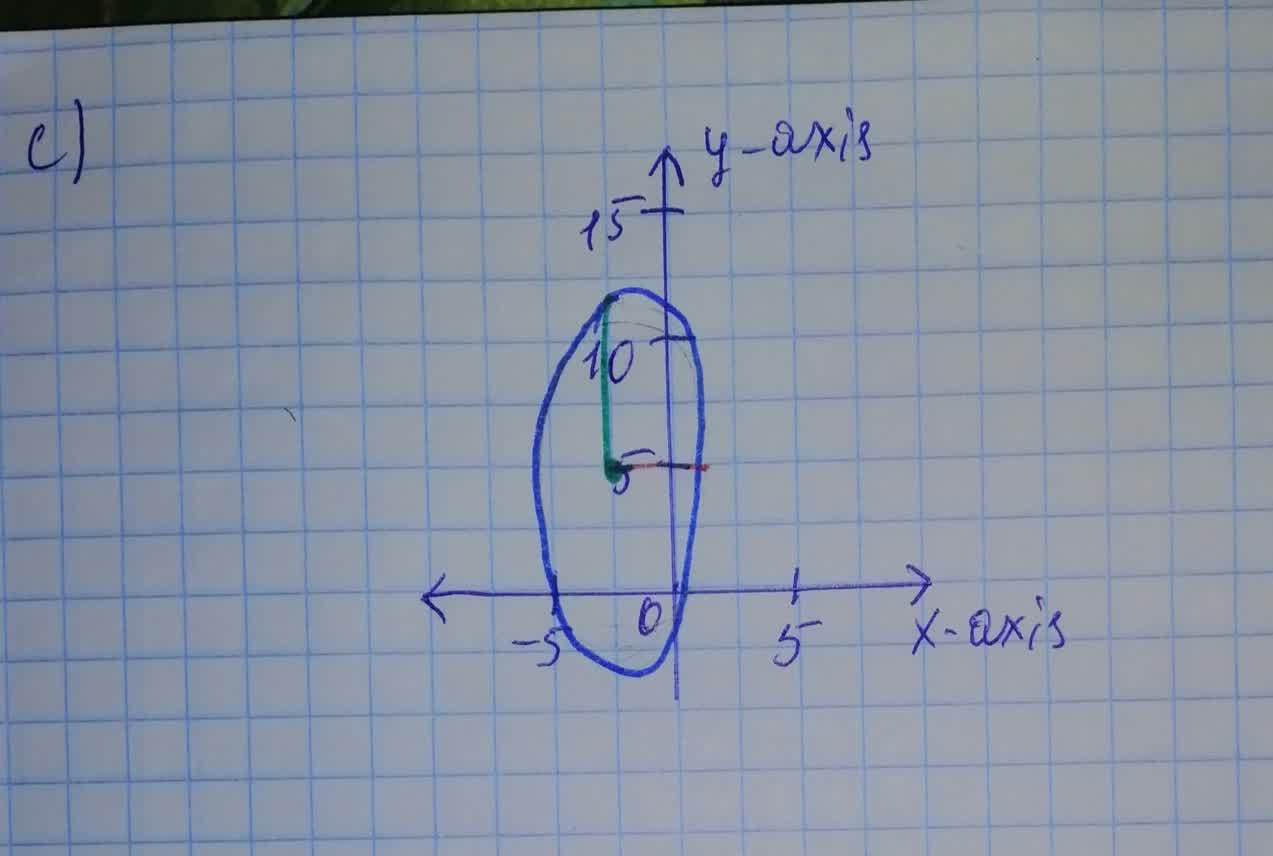Show that the equation represents a conic section (a) x^2 – 2x – 4y^2 – 12y = -8 (b) 2x^2 + 4x - 5y + 7 = 0 (c) 8a^2 + 8x + 2y^2 – 20y = 12bobbie71G 2021-08-09 Answered
Show that the equation represents a conic section. Sketch the conic section, and indicate all pertinent information (such as foci, directrix, asymptotes, and so on). $$\displaystyle{\left({a}\right)}{x}^{{2}}–{2}{x}–{4}{y}^{{2}}–{12}{y}=-{8}{\left({b}\right)}{2}{x}^{{2}}+{4}{x}-{5}{y}+{7}={0}{\left({c}\right)}{8}{a}^{{2}}+{8}{x}+{2}{y}^{{2}}–{20}{y}={12}$$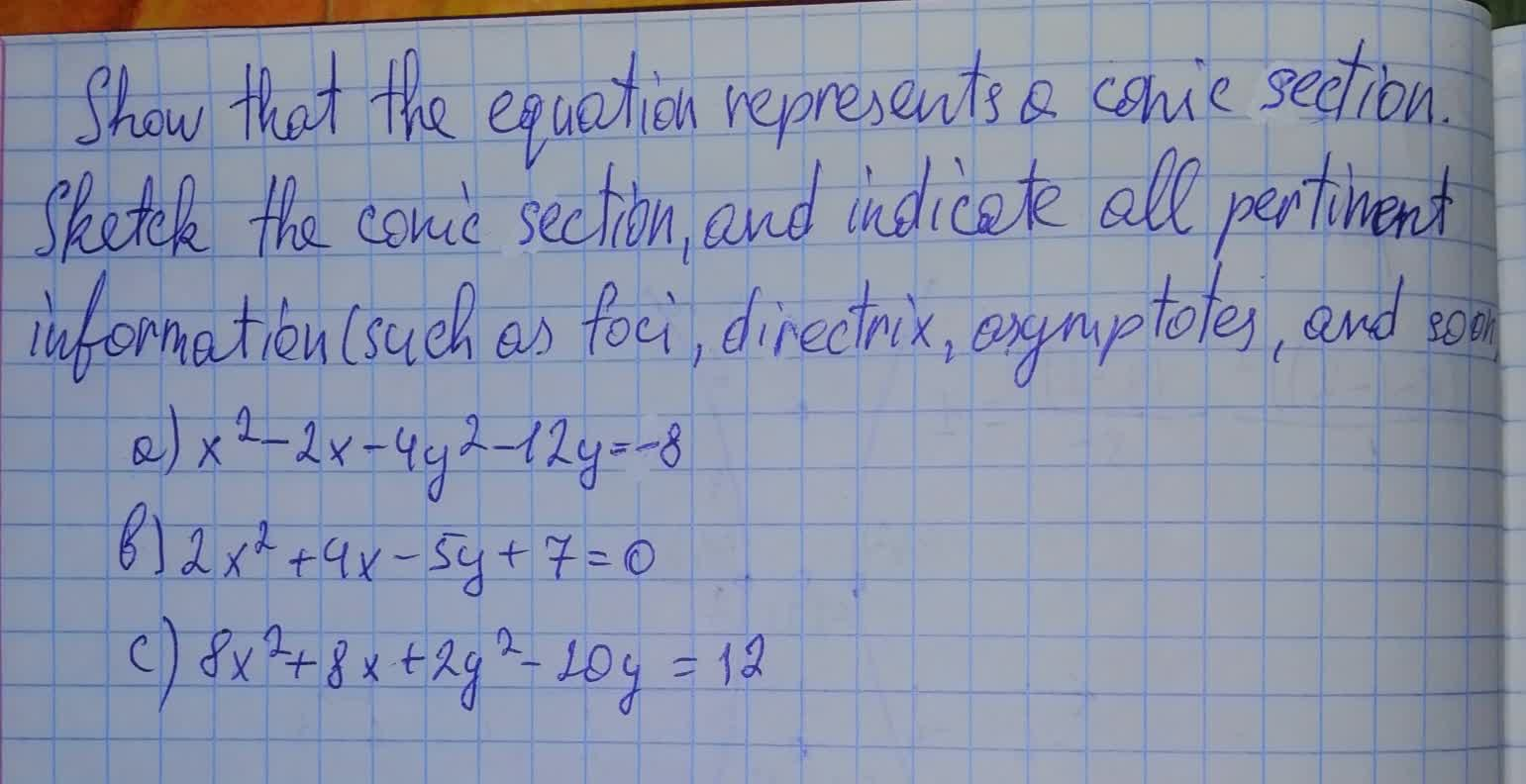• Questions are typically answered in as fast as 30 minutes

Solve your problem for the price of one coffee

• Math expert for every subject
• Pay only if we can solve itJozlyn